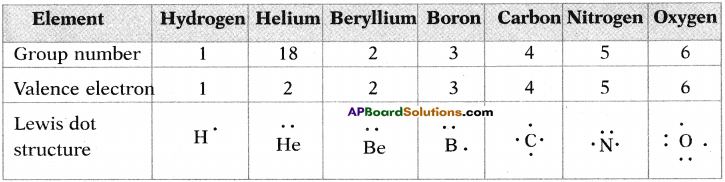# AP SSC 10th Class Chemistry Solutions Chapter 10 Chemical Bonding

AP State Board Syllabus AP SSC 10th Class Chemistry Solutions Chapter 10 Chemical Bonding Textbook Questions and Answers.

## AP State Syllabus SSC 10th Class Chemistry Solutions 10th Lesson Chemical Bonding

### 10th Class Chemistry 10th Lesson Chemical Bonding Textbook Questions and Answers

Question 1.
List the factors that determine the type of bond that will be formed between two atoms. (AS1)
(OR)
How can you identify the type of bond formation between two atoms?

• The strength of attraction or repulsion between atoms.
• Electrons in valence shell (valence electrons).

Question 2.
Explain the difference between the valence electrons and the covalency of an element. (AS1)
(OR)
How are valence electrons different from the covalency of element? Explain with examples.
Valence electrons :

• Number of electrons in the outermost orbit or an atom is called its valence electrons.
• Ex: Na (Z = 11). It has 2e in I orbit, 8e in II orbit and 1e in III orbit.
• So number of valence electrons in Na atom are ‘l’.

Covalency of an element:

• Number of valance electrons which are taking part in covalent bond is called covalency.
• The electron configuration of Boran is 1s² 2s² 2p¹.
• It has three valance electrons.
• So its covalency is 3.Question 3.
A chemical compound has the following Lewis notation : (AS1)
a) How many valence electrons does element Y have?
b) What is the valency of element Y?
c) What is the valency of element X?
d) How many covalent bonds are there in the molecule?
e) Suggest a name for the elements X and Y.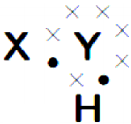a) 6
b) 2
c) 1
d) two
e) X – is hydrogen and Y – is oxygen. The formed molecule is H2O.

Question 4.
Why do only valence electrons involve in bond formation? Why not electron of inner shells? Explain. (AS1)
(OR)
Which shell electrons involve in bond formation? Explain. What is the reason behind it?

• The nucleus and the electrons in the inner shell remain unaffected when atoms come close together.
• But the electrons in the outermost shell (valence shell) of atoms get affected.
• The inner shell electrons are strongly attracted by the nucleus when compared to the valence electrons.
• So electrons in valence shell (valence electrons) are responsible for the formation of bond between atoms.Question 5.
Explain the formation of sodium chloride and calcium oxide on the basis of the concept of electron transfer from one atom to another atom. (AS1)
(OR)
Explain the formation of any two compounds according to Kossel’s theory.
I. Formation of sodium chloride (NaCl) :
1) Sodium chloride is formed from the elements sodium (Na) and chlorine (Cl).

2) Cation formation:
i) When sodium (Na) atom loses one electron to get octet electron configuration, it forms a cation (Na+).
ii) Now Na+ gets electron configuration that of Neon (Ne) atom.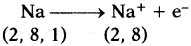3) Anion Formation :
i) Chlorine has shortage of one electron to get octet in its valence shell.
ii) So it gains the electron that was lost by Na to form anion and gets electron configuration of Argon (Ar).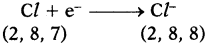4) Formation of NaCl :
i) Transfer of electrons between ‘Na’ and ‘Cl’ atoms, results in the formation of ‘Na+‘ and ‘Cl’ ions.
ii) These oppositely charged ions get attracted towards each other due to electrostatic forces and form the compound sodium chloride (NaCl).
Na+(g) + Cl(g) → Na+Cl(s) or NaCl

II. Formation of calcium oxide (CaO) :
1. Calcium (Ca) reacts with oxygen (0) to form an ionic compound calcium oxide (CaO).

2. Atomic number of Calcium is 20. Its electronic configuration is 2, 8, 8, 2.
3.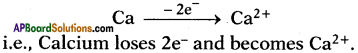4. Atomic number of Oxygen is 8. Its electronic configuration is 2, 6.

5.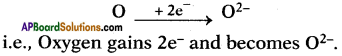6. These oppositely charged ions get attracted towards each other due to electrostatic forces and form the compound calcium oxide (CaO).
Ca2+ + O2- → Ca2+O2- (or) CaOQuestion 6.
A, B and C are three elements with atomic number 6, 11 and 17 respectively.
i) Which of these cannot form ionic bond? Why? (AS1)
ii) Which of these cannot form covalent bond? Why? (AS1)
iii) Which of these can form ionic as well as covalent bonds? (AS1)
i) ‘A’ cannot form ionic bond. Its valence electrons are 4. It is difficult to lose or gain 4e to get octet configuration. So it forms covalent bond [Z of A is 6 so it is carbon (C)].

ii) ‘B’ cannot form covalent bond. Its valence electrons are 1 only. So it is easy to donate for other atom and become an ion. So it can form ionic bond [Z of B is 11, so it is sodium (Na)].

iii) Element C can form ionic as well as covalent bonds. Atomic number of Cl is 17. It is able to participate with Na in ionic bond and with hydrogen in HCl molecule as covalent bond.

Question 7.
How do bond energies and bond lengths of molecule help us in predicting their chemical properties? Explain with examples. (AS1)
(OR)
How can you explain with examples that bond energies and bond lengths are used to recognise chemical properties?
1. Bond length :
Bond length or bond distance is the equilibrium distance between the nuclei of two atoms which form a covalent bond.

2. Bond energy :
Bond energy or bond dissociation energy is the energy needed to break a covalent bond between two atoms of a diatomic covalent compound in its gaseous state.

3. If the nature of the bond between the same two atoms changes the bond length also changes. For example, the bond lengths between two carbon atoms are C – C > C = C > C = C.

4. Thus the various bond lengths between the two carbon atoms are in ethane 1.54 Å, ethylene 1.34 Å, acetylene 1.20 Å.

5. The bond lengths between two oxygen atoms are in H2O2 (O – O) is 1.48 Å and in O2 (O = O) is 1.21 Å.
6. Observe the table.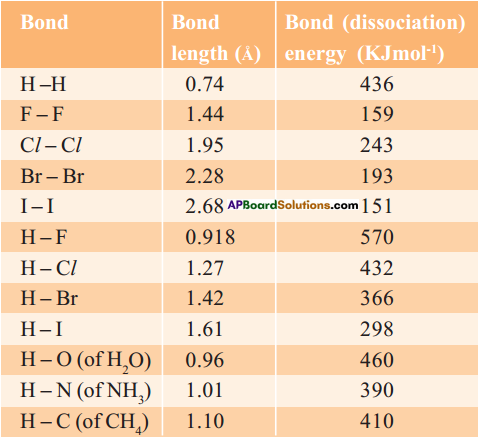7. When bond length decreases, then bond dissociation energy increases.

8. When bond length increases, then bond dissociation energy decreases.

9. Bond length of H – H in H2 molecule is 0.74 Å and its bond dissociation energy is 436 KJ/mol, whereas bond length of F – F in F2 molecule is 1.44 Å and its bond dissociation energy is 159 KJ/mol.

10. Melting and boiling points of substances also can be determined by this bond energies and bond lengths.

Question 8.
Predict the reasons for low melting point for covalent compounds when compared with ionic compounds. (AS2)
(OR)
“Covalent compounds have low melting point.” What Is the reason for this statement? Explain.
They are covalent compounds.

• The melting point is low due to the weak Vander Waal’s forces of attractions between the covalent molecules.
• The force of attraction between the molecules of a covalent compound is very weak.
• Only a small amount of heat energy is required to break these weak molecular forces, due to which covalent compounds have low melting points and low boiling points.
• Please note that some of the covalent solids like diamond and graphite have, however very high melting points and boiling points.Question 9.
Collect the information about properties and uses of covalent compounds and prepare a report. (AS4)
(OR)
Generally these compounds are non-polar in nature. What are those compounds? Explain their properties and uses.
(OR)
Write any two uses and two properties of covalent compounds.
The compounds are covalent.
Properties of covalent compounds :

1. Covalent compounds are usually liquids or gases, only some of them are solids.
2. The covalent compounds are usually liquids or gases due to the weak force of attraction between their molecules.
3. Covalent compounds have usually low melting and low boiling points.
4. Covalent compounds are usually in soluble in water but they are soluble in organic solvents.
5. Covalent compounds do not conduct electricity.

Uses of covalent compounds :

1. Covalent compounds form 99% of our body.
2. Water is a covalent compound. We know its many uses.
3. Sugars, food substances, tea and coffee are all covalent compounds.
4. Air we breathe in contains covalent molecules of oxygen and nitrogen.
5. Almost everything on earth other than most simple in organic salts are covalent.

Question 10.
Draw simple diagrams to show how electrons are arranged in the following covalent molecules : (AS5)
a) Calcium oxide (CaO)
b) Water (H2O)
c) Chlorine (Cl2)
a) Calcium oxide (CaO) :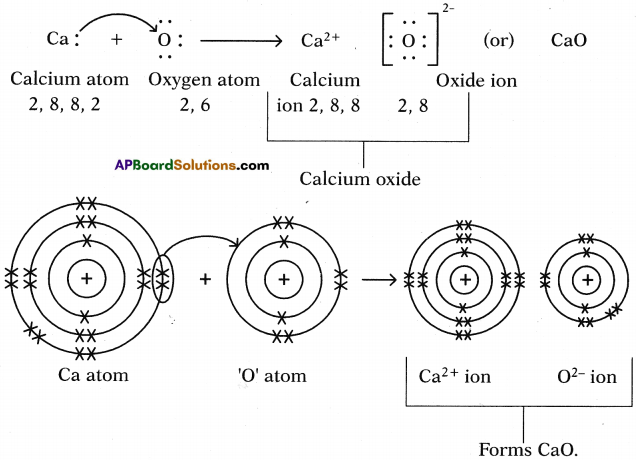b) Water (H2O):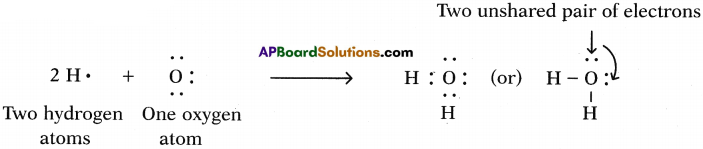The formation of water molecule can be shown like this also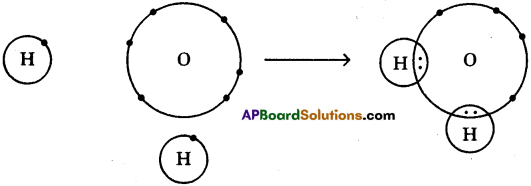c) Chlorine (Cl2):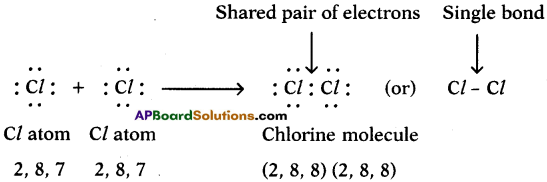We can explain the formation of Cl2 molecule in this way also.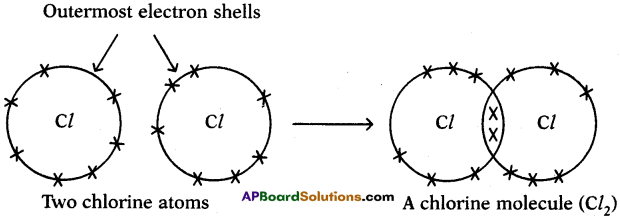Question 11.
Represent the molecule H2O using Lewis notation. (AS5)
(OR)
How can you explain the formation of H2O molecule using dot structure?
One atom of oxygen shares its two electrons with two hydrogen atoms to form a water molecule.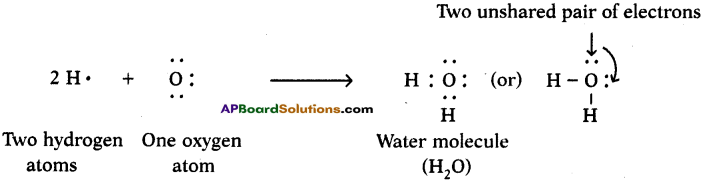Question 12.
Represent each of the following atoms using Lewis notation : (AS5)
a) Beryllium
b) Calcium
c) Lithium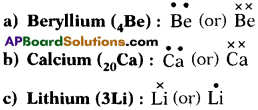Question 13.
Represent each of the following molecules using Lewis notation : (AS5)
a) Bromine gas (Br2)
b) Calcium chloride (CaCl2)
c) Carbon dioxide (CO2)
d) Which of the three molecules listed above contains a double bond?
a) Bromine gas (Br2) :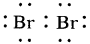b) Calcium chloride (CaCl2)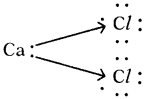c) Carbon dioxide (CO2) :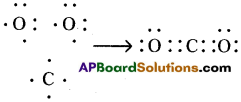d) CO2, contains double bond in above list. Its structure is like this : O = C = O.Question 14.
Two chemical reactions are described below. (AS5)
♦ Nitrogen and hydrogen react to form ammonia (NH3).
♦ Carbon and hydrogen bond to form a molecule of methane (CH4).
For each reaction give :
a) The valency of each of the atoms involved in the reaction.
b) The Lewis structure of the product that is formed.
a) ♦ Nitrogen and hydrogen react to form ammonia (NH3):
i) The valency of nitrogen is 3 and hydrogen is 1.
ii) The chemical formula of the product is NH3

♦ Carbon and hydrogen bond to form a molecule of methane (CH4):
i) The valency of carbon is 4 and hydrogen is 1.
ii) The chemical formula of the product is CH4.

b) ♦ The Lewis structure of the product that is formed (: NH3)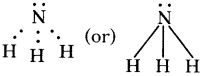♦ The Lewis structure of the product that is formed (CH4)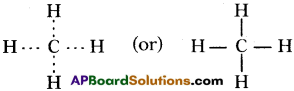Question 15.
How does Lewis dot structure help in understanding bond formation between atoms? (AS6)
(OR)
What is the use of Lewis dot structure in bond formation? Explain.

1. Only the outermost electrons of an atom take part in chemical bonding.
2. They are known as valence electrons.
3. The valence electrons in an atom are represented by putting dots (•) on the symbol of the element, one dot for each valence electron.
4. For example, sodium atom has 1 valence electron in its outermost shell, so we put 1 dot with the symbol of sodium and write Na• for it.
5. Sodium atom loses this 1 electron to form sodium ion.
6. By knowing the valence electrons of two different atoms by Lewis dot structure, we can understand which type of bond is going to establish between them and forms corresponding molecule.

Question 16.
What is octet rule? How do you appreciate role of the ‘octet rule’ in explaining the chemical properties of elements? (AS6)
(OR)
Which rule decides whether given element is chemically stable or not? Appreciate that rule.
Octet rule decides whether given element is stable or not.
Octet rule :

• ‘The atoms of elements tend to undergo chemical changes that help to leave their atoms with eight outer shell electrons.”
• It was found that the elements which participate in chemical reaction get octet (or) ns2 np6 configuration similar to that of noble gas elements.

Role of octet in chemical properties of elements :

1. Except He remaining inert gas elements have 8 electrons in their outermost orbit. Since these elements are having stable octet configuration in their outermost orbit, they are very stable.
2. They do not allow the outermost electrons to take part in chemical reactions.
3. So by having octet configuration for these elements we can conclude these are chemically inertial.
4. If any group of elements (take halogens) which contain 7 electrons in their outermost orbit, they require only 1 e to get octet configuration.
5. So they try to participate in chemical reaction to get that 1 difference electron for octet configuration.
6. Similarly, Na contains 2, 8, 1 as its electronic configuration.
7. So it loses le from its outermost shell; it should have 8e in its outer shell and get the octet configuration.
8. Thus the octet rule helps in explaining the chemical properties of elements.Question 17.
Explain the formation of the following molecules using valence bond theory.
a) N2 molecule
b) O2 molecule
(OR)
Write the formation of double bond and triple bond according to valence bond theory.
(OR)
Who proposed Valence Bond Theory? Explain the formation of N2 molecule by using this theory.
Linus Pauling was proposed valence bond theory.
Formation of N2 molecule :

1. Electronic configuration of Nitrogen is 1s² 2s² 2px¹ 2py¹ 2pz¹.
2. Suppose that px orbital of one Nitrogen atom overlaps the px orbital of other ‘N’ atom giving σ px – px bond along the inter nuclear axis.
3. The py and pz orbitals of one ‘N’ atom overlaps with the py and pz orbital of other ‘N’ atom laterally giving π py – py and π pz – pz bonds.
4. Therefore, N2 molecule has a triple bond between two Nitrogen atoms.Formation of O2 molecule :

1. Electronic configuration of ‘O’ is 1s² 2s² 2px² 2py¹ 2pz¹.
2. If the Py orbital of one ‘O’ atom overlaps the py orbital of other ‘O’ atom along inter- nuclear axis, a σ py – py bond is formed.
3. pz orbital of oxygen atom overlaps laterally, perpendicular to inter nuclear axis giving a π py – pz bond.
4. So O2 molecule has a double bond between the two oxygen atoms.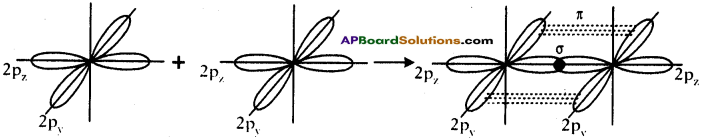Question 18.
What is hybridisation? Explain the formation of the following molecules using hybridisation.
a) BeCl2
b) BF3
Explain the formation of sp and sp² hybridisation using examples.
(OR)
What is the name given to inter mixing of atomic orbitals to form new orbitals. Explain the formation of following molecules by using that process,
a) BeCl2
b) BF3
This process is called hybridisation.
Hybridisation :
It is a phenomenon of inter mixing of atomic orbitals of almost equal energy which are present in the outer shells of the atom and their reshuffling or redistribution into the same number of orbitals but with equal properties like energy and shape.

a) Formation of BeCl2 (Beryllium chloride) molecule :

1. 4Be has electronic configuration 1s² 2s².
2. It has no unpaired electrons.
3. It is expected not to form covalent bonds, but informs two covalent bonds one each with two chlorine atoms. „
4. To explain this, an excited state is suggested for Beryllium in which an electron from ‘2s’ shifts to 2px level.
5. Electronic configuration of 4Be is 1s² 2s¹ 2px¹].
6. Electronic configuration of 17Cl is 1s² 2s² 2p6 3s² 3px² 3py² 3pz¹.
7. If Be forms two covalent bonds with two chlorine atoms, one bond should be σ 2s-3p due to the overlap of ‘2s’ orbital of Be, the ‘3pz‘ orbital of one chlorine atom.
8. The other bond should be σ 2p-3p due to the overlap of ‘2px’ orbital of Be atom the 3p orbital of the other chlorine atom.
9. As the orbitals overlapping are different, the bond strengths of two Be-Cl must be different.
10. But, both bonds are of same strength and Cl$$\hat{\mathrm{Be}}$$ Cl is 180°.

The Hybridisation of BeCl2 can be explained in this way also :
a) Be atom in its excited state allows its 2s orbital and 2px orbital which contain unpaired electrons to intermix and redistribute to two identical orbitals.
b) As per Hund’s rule each orbital gets one electron.
c) The new orbitals based on the types of orbitals that have undergone hybridisation are called sp orbitals.
d) The two sp orbitals of Be get separated by 180°.
e) Now each chlorine atom comes with its 3pz¹ orbital and overlaps it the sp orbitals of Be forming two identical Be-Cl bonds (σ sp-p bonds).
Cl$$\hat{\mathrm{Be}}$$ Cl = 180°.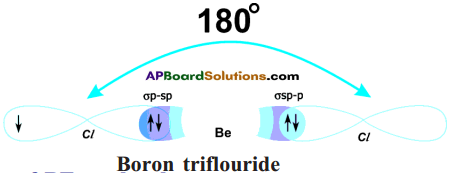f) Both the bonds are of same strength.

b) Formation of BF3 molecule :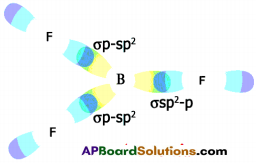1. 5B has electronic configuration 1s² 2s² 2pxh
2. The excited electronic configuration of 5B is 1s² 2s¹ 2px¹ 2py¹
3. As it forms three identical B-F bonds in BF3.
4. It is suggested that excited ‘B’ atom undergoes hybridisation.
5. There is an intermixing of 2s, 2px, 2py orbitals and their redistribution into three identical orbitals called sp² hybrid orbitals.
6. For three sp² orbitals to get separated to have minimum repulsion the angle between any two orbitals is 120° at the central atom and each sp² orbital gets one election.
7. Now three fluorine atoms overlap their 2pz orbitals containing unpaired electrons (F9 1s² 2s² 2px² 2py² 2pz¹) the three sp² orbitals of ‘B’ that contain unpaired electrons to form three σsp²-p bonds.

Fill in the Blanks

1. Electrons in the outermost orbit are called …………………… .
2. Except …………………… gas all other noble gases have octet in their valence shell.
3. Covalency of elements explains about member of …………………… formed by the atom.
4. Valence bond theory was proposed by …………………… .
5. In …………………… bonding the valence electrons are shared among all the atoms of the metallic elements.

1. valence electrons
2. Helium
3. covalent bonds
4. Linus Pauling
5. covalent

Multiple Choice Questions

1. Which of the following elements is electronegative?
A) Sodium
B) Oxygen
C) Magnesium
D) Calcium
B) Oxygen

2. An element 11X23 forms an ionic compound with another element ‘Y’. Then the charge on the ion formed by X is
A) +1
B) +2
C) -l
D) – 2
A) +13. An element ‘A’ forms a chloride ACl4. The number of electrons in the valence shell of ‘A’
A) 1
B) 2
C) 3
D) 4
D) 4

### 10th Class Chemistry 10th Lesson Chemical Bonding InText Questions and Answers

10th Class Chemistry Textbook Page No. 153

Question 1.
How do elements usually exist?
They may exist as a single atom or as a group of atoms.

Question 2.
Do atoms exist as a single atom or as a group of atoms?
Atoms exist as a single atom, sometimes as a group of atoms also.Question 3.
Are there elements which exist as atoms?
Yes. There are elements which exist as atoms.

Question 4.
Why do some elements exist as molecules and some as atoms?
By following different laws of chemical combination the chemical compounds take place as a result of combination of atoms of various elements in different ways.

Question 5.
Why do some elements and compounds react vigorously while others are inert?
1) Number of electrons in their outermost shell.
2) Bond strength between the atoms in compound.

Question 6.
Why is the chemical formula for water H2O and for sodium chloride NaCl, why not HO2 and NaCl2?
Answee:
Valencies of the atoms participating in the molecules.

Question 7.
Why do some atoms combine dille tl do not?
1) Atoms which have 8e“ in their outer shell will not combine.
2) Atoms which have more than or less than 8e“ in their outer shell will combine.

Question 8.
Are elements and compounds simply made up of separate atoms Individually arranged?
No. Elements and compounds are not simply made up of separate atoms individually arranged.Question 9.
Is there any attraction between atoms?
Yes. There is some attraction betwen atoms.

Question 10.
What is that holding them together?
Force of attraction between them.

10th Class Chemistry Textbook Page No. 155

Question 11.
Why is there absorption of energy in certain chemical reactions and release of energy in other reactions?
Because of bond energy between the atoms in a molecule.

Question 12.
Where does the absorbed energy go?
For breaking chemical bonds between atoms in a molecule.

Question 13.
Is there any relation to energy and bond formation between atoms?
Yes. There is some relation to energy and bond formation between atoms.Question 14.
What could be the reason for the change in reactivity of elements?
Number of electrons in their outermost orbit.

Question 15.
What could be the reason for this?
They have 8 (e) electrons in their outermost orbit.

10th Class Chemistry Textbook Page No. 157

Question 16.
What did you notice in Lewis dot structure of noble gases and electronic configurations of the atoms of these elements shown in table – 1?
Except He remaining Ne, Ar, Kr have 8 electrons in their outermost orbit.

10th Class Chemistry Textbook Page No. 158

Question 17.
What have you observed from the above conclusions about the main groups?

1. Number of gained electrons of non-metals in their valency.
2. Number of lost electrons of metals in their valency.Question 18.
Why do atoms of elements try to combine and form molecules?
To attain stable electronic configuration.

10th Class Chemistry Textbook Page No. 159

Question 19.
Is it accidental that IA to VIIA main group elements during chemical reactions get eight electrons in the outermost shells of their ions, similar to noble gas atoms?
No, it cannot be simply accidental.

Question 20.
Explain the formation of ionic compounds NaCl, MgCl2, Na2O and AlCl3 through Lewis electron dot symbols (formulae).
1) Lewis electron dot symbol for NaCl: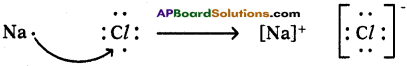Formation of sodium chloride (NaCl) :
Sodium chloride is formed from the elements sodium and chlorine. It can be explained as follows.
Na(s) + ½Cl2(g) → NaCl2

Cation formation :
When sodium (Na) atom loses one electron to get octet electron configuration it forms a cation (Na+) and gets electron configuration that of Neon (Ne) atom.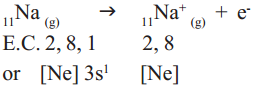Anion formation :
Chlorine has shortage of one electron to get octet in its valence shell. So it gains the electron from Na atom to form anion and gets electron configuration as that of argon (Ar).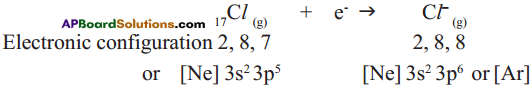Formation of the compound NaCl from its ions :
Transfer of electrons between ‘Na’ and ‘Cl’ atoms, results in the formation of ‘Na+‘ and ‘Cl‘ ions. These oppositely charged ions get attracted towards each other due to electrostatic forces and form the compound sodium chloride (NaCl).
Na+(g) + Cl(g) → Na+Cl(s) or NaCl

2) Lewis electron dot symbol for MgCl2:
MgCl2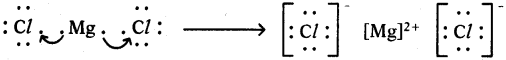Formation of magnesium chloride (MgCl2):
Magnesium chloride is formed from the elements magnesium and chlorine. The bond formation MgCl2 in brief using chemical equation is as follows :
Mg(s) + Cl2(g) → MgCl2(g)
Cation formation: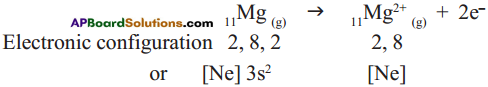Anion formation :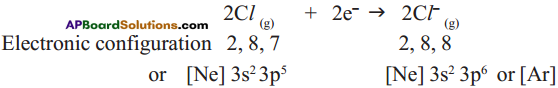The compound MgCl2 formation from its ions :
Mg2+ gets ‘Ne’ configuration and
Each Cl gets ‘Ar’ configuration
Mg2+(g) + 2 Cl(g) → MgCl2(s)
One ‘Mg’ atom transfers two electrons one each to two ‘Cl’ atoms and so formed Mg2+ and 2Cl attract to form MgCl2.

3) Lewis electron dot symbol for (Na2O) :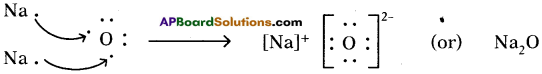Formation of di sodium monoxide (Na2O):
Di sodium monoxide formation can be explained as follows:
Cation formation (Na+ formation):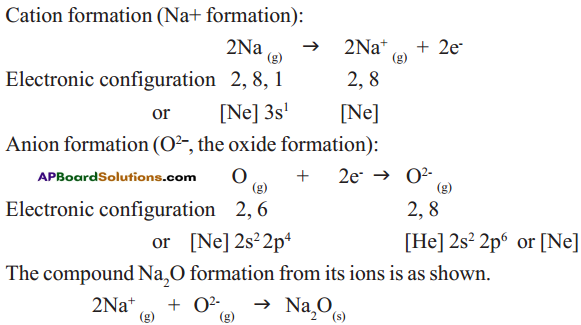Two ‘Na’ atoms transfer one electron each to one oxygen atom to form 2 Na+ and O2-
Each Na+ gets ‘Ne’ configuration and O2- gets ‘Ne’ configuration.
These ions (2Na+ and O2-) attract to form Na2O.

4) Lewis electron dot symbol for (AlCl3):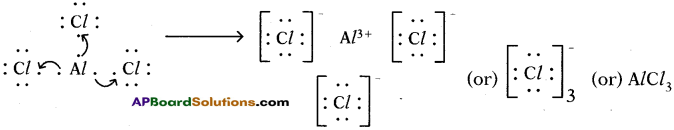Formation of aluminium chloride (AlCl3):
Aluminium chloride formation can be explained as follows:
Formation of aluminium ion (Al3+), the cation: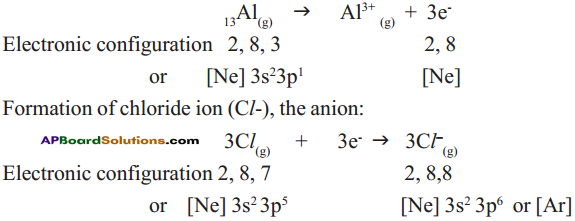Each aluminium atom loses three electrons and three chlorine atoms gain them, one electron each.
The compound AlCl3 is formed from its component ions by the electrostatic forces of attractions.
Al3+(g) + 3 Cl(g) → AlCl3(s)

10th Class Chemistry Textbook Page No. 163

Question 21.
How do cations and anions of an ionic compound exist in its solid state?
Ionic compounds exist in crystalline state.

Question 22.
Do you think that pairs of Na+ Cl as units would be present in the solid crystal?
Yes. I think that pairs of Na+ Cl as units would be present in the solid crystal.

10th Class Chemistry Textbook Page No. 164

Question 23.
Can you explain the reasons for all these?
Ionic bond is formed between atoms of elements with electronegativity, difference equal to or greater than 1.9.

10th Class Chemistry Textbook Page No. 165

Question 24.
Can you say what type of bond exists between atoms of nitrogen molecule?
Triple bond exists between atoms of nitrogen molecule.

10th Class Chemistry Textbook Page No. 168

Question 25.
What do you understand from bond lengths and bond energies?
Bond lengths and bond energies are not same when the atoms that form the bond are different.Question 26.
Are the values not different for the bonds between different types of atoms?
Yes. The values are not different for the bonds between different types of atoms.

10th Class Chemistry Textbook Page No. 170

Question 27.
What is the bond angle in a molecule?
It is the angle subtended by two imaginary lines that pass from the nuclei of two atoms which form the covalent bonds with the central atom through the nucleus of the central atom at the central atom.

10th Class Chemistry Textbook Page No. 172

Question 28.
How is MCI molecule formed?